# TFS 0.X[LUA]Boss Room

#### potinho

##### Intermediate OT User
Hello how are you?

I have this script that I copied from anihilator and I'm using it as a boss room, it works great for 4 players. However, I would like to increase the number of tiles, but it would still be possible to enter the room with any amount of players (4 or more), could you help me to fix the code for this?

My actual boss room: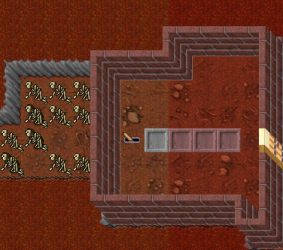I pretend to use like this, but still goes with 4, 5, 6, 7 or 8 players.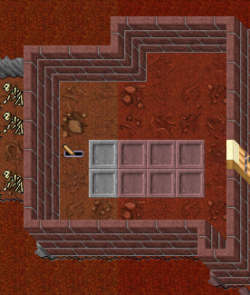This my actual script
Lua:
``````function onUse(cid, item, frompos, item2, topos)
if item.uid == 9102 then
local count = 0
local config = {
questLevel = 100,
playerPositions = {
{start = {x=1893, y=447, z=11}, destination = {x=1801, y=472, z=9}},
{start = {x=1894, y=447, z=11}, destination = {x=1802, y=472, z=9}},
{start = {x=1895, y=447, z=11}, destination = {x=1803, y=472, z=9}},
{start = {x=1896, y=447, z=11}, destination = {x=1804, y=472, z=9}}
},
spawnMonsters = {
{position = {x=1812, y=452, z=9}, monster = "Ulquiorra"}
}
}
local players = {}
for i = 1, #config.playerPositions do
local pid = getTopCreature(config.playerPositions[i].start)
if pid.uid > 0 then
if isPlayer(pid.uid) then
if getPlayerLevel(pid.uid) >= config.questLevel then
table.insert(players, pid.uid)
end
end
end
end
if #players == #config.playerPositions then
for i = 1, #players do
doTeleportThing(players[i], config.playerPositions[i].destination)
doSendMagicEffect(config.playerPositions[i].destination, CONST_ME_TELEPORT)
end
for v = 1, #config.spawnMonsters do
doSummonCreature(config.spawnMonsters[v].monster, config.spawnMonsters[v].position)
doSendMagicEffect(config.spawnMonsters[v].position, CONST_ME_TELEPORT)
end
else
doPlayerSendCancel(cid, "Sorry, not possible.")
end
return true
end
end``````

Solution
Works, thanks Xiki! To remove the logs, just delete "print" lines, right?
correct for the most part. xP
Lua:
``````local config = {
questLevel = 100,
minimumPlayersRequired = 4,
playerPositions = {
{start = {x=1893, y=447, z=11}, destination = {x=1801, y=472, z=9}},
{start = {x=1894, y=447, z=11}, destination = {x=1802, y=472, z=9}},
{start = {x=1895, y=447, z=11}, destination = {x=1803, y=472, z=9}},
{start = {x=1896, y=447, z=11}, destination = {x=1804, y=472, z=9}},
{start = {x=1893, y=448, z=11}, destination = {x=1801, y=473, z=9}},
{start = {x=1894, y=448, z=11}, destination = {x=1802, y=473, z=9}},
{start = {x=1895, y=448, z=11}, destination = {x=1803, y=473...``````
like edit playerPositions and done

like edit playerPositions and done
it well be possible do the quest with 4, 5, 6, 7 or 8 players? i dont wanna fix the amount of players, now if i try alone, shows message "its not possible"

Yeah it will teleport only the players on tiles, no matters if its 1 or 8 players
Lua:
``````playerPositions = {
{start = {x=1893, y=447, z=11}, destination = {x=1801, y=472, z=9}},
{start = {x=1894, y=447, z=11}, destination = {x=1802, y=472, z=9}},
{start = {x=1895, y=447, z=11}, destination = {x=1803, y=472, z=9}},
{start = {x=1896, y=447, z=11}, destination = {x=1804, y=472, z=9}},
{start = {x=1893, y=448, z=11}, destination = {x=1801, y=473, z=9}},
{start = {x=1894, y=448, z=11}, destination = {x=1802, y=473, z=9}},
{start = {x=1895, y=448, z=11}, destination = {x=1803, y=473, z=9}},
{start = {x=1896, y=448, z=11}, destination = {x=1804, y=473, z=9}}
},``````

Yeah it will teleport only the players on tiles, no matters if its 1 or 8 players
Lua:
``````playerPositions = {
{start = {x=1893, y=447, z=11}, destination = {x=1801, y=472, z=9}},
{start = {x=1894, y=447, z=11}, destination = {x=1802, y=472, z=9}},
{start = {x=1895, y=447, z=11}, destination = {x=1803, y=472, z=9}},
{start = {x=1896, y=447, z=11}, destination = {x=1804, y=472, z=9}},
{start = {x=1893, y=448, z=11}, destination = {x=1801, y=473, z=9}},
{start = {x=1894, y=448, z=11}, destination = {x=1802, y=473, z=9}},
{start = {x=1895, y=448, z=11}, destination = {x=1803, y=473, z=9}},
{start = {x=1896, y=448, z=11}, destination = {x=1804, y=473, z=9}}
},``````
not work, expect 8 players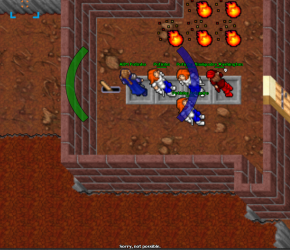#### Attachments

Lua:
``````function onUse(cid, item, frompos, item2, topos)
if item.uid == 9102 then
local config = {
questLevel = 100,
playerPositions = {
{start = {x=1893, y=447, z=11}, destination = {x=1801, y=472, z=9}},
{start = {x=1894, y=447, z=11}, destination = {x=1802, y=472, z=9}},
{start = {x=1895, y=447, z=11}, destination = {x=1803, y=472, z=9}},
{start = {x=1896, y=447, z=11}, destination = {x=1804, y=472, z=9}}
},
spawnMonsters = {
{position = {x=1812, y=452, z=9}, monster = "Ulquiorra"}
}
}
local players = {}
for i = 1, #config.playerPositions do
local topPlayer = getTopCreature(config.playerPositions[i].start)
if topPlayer and isPlayer(topPlayer) and getPlayerLevel(topPlayer) >= config.questLevel then
players[#players + 1] = topPlayer.uid
end
end

if #players == 0 then
doPlayerSendCancel(cid, "Sorry, not possible.")
return false
end

for i = 1, #players do
doSendMagicEffect(getThingPosition(players[i]), CONST_ME_POFF)
doTeleportThing(players[i], config.playerPositions[i].destination)
doSendMagicEffect(getThingPosition(players[i]), CONST_ME_TELEPORT)
end

for i = 1, #config.spawnMonsters do
doSummonCreature(config.spawnMonsters[i].monster, config.spawnMonsters[i].position)
doSendMagicEffect(config.spawnMonsters[i].position, CONST_ME_TELEPORT)
end
end
return true
end``````

Edited - try again

Last edited:
Lua:
``````function onUse(cid, item, frompos, item2, topos)
if item.uid == 9102 then
local config = {
questLevel = 100,
playerPositions = {
{start = {x=1893, y=447, z=11}, destination = {x=1801, y=472, z=9}},
{start = {x=1894, y=447, z=11}, destination = {x=1802, y=472, z=9}},
{start = {x=1895, y=447, z=11}, destination = {x=1803, y=472, z=9}},
{start = {x=1896, y=447, z=11}, destination = {x=1804, y=472, z=9}}
},
spawnMonsters = {
{position = {x=1812, y=452, z=9}, monster = "Ulquiorra"}
}
}
local players = {}
for i = 1, #config.playerPositions do
local topPlayer = getTopCreature(config.playerPositions[i].start)
if topPlayer and isPlayer(topPlayer) and getPlayerLevel(topPlayer) >= config.questLevel then
players[#players + 1] = topPlayer
end
end

if #players == 0 then
doPlayerSendCancel(cid, "Sorry, not possible.")
return false
end

for i = 1, #players do
doSendMagicEffect(getThingPosition(players[i]), CONST_ME_POFF)
doTeleportThing(players[i], config.playerPositions[i].destination)
doSendMagicEffect(getThingPosition(players[i]), CONST_ME_TELEPORT)
end

for i = 1, #config.spawnMonsters do
doSummonCreature(config.spawnMonsters[i].monster, config.spawnMonsters[i].position)
doSendMagicEffect(config.spawnMonsters[i].position, CONST_ME_TELEPORT)
end
end
return true
end``````
push lever, but nothing happens. No error in console.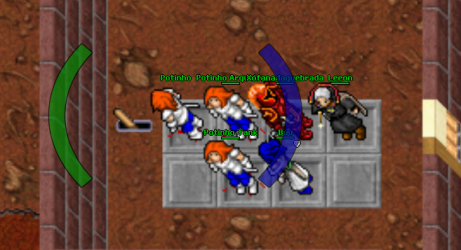Post automatically merged:

my actual script

Lua:
``````function onUse(cid, item, frompos, item2, topos)
if item.uid == 9102 then
local config = {
questLevel = 100,
playerPositions = {
{start = {x=1893, y=447, z=11}, destination = {x=1801, y=472, z=9}},
{start = {x=1894, y=447, z=11}, destination = {x=1802, y=472, z=9}},
{start = {x=1895, y=447, z=11}, destination = {x=1803, y=472, z=9}},
{start = {x=1896, y=447, z=11}, destination = {x=1804, y=472, z=9}},
{start = {x=1893, y=448, z=11}, destination = {x=1801, y=473, z=9}},
{start = {x=1894, y=448, z=11}, destination = {x=1802, y=473, z=9}},
{start = {x=1895, y=448, z=11}, destination = {x=1803, y=473, z=9}},
{start = {x=1896, y=448, z=11}, destination = {x=1804, y=473, z=9}}
},
spawnMonsters = {
{position = {x=1812, y=452, z=9}, monster = "Ulquiorra"}
}
}
local players = {}
for i = 1, #config.playerPositions do
local topPlayer = getTopCreature(config.playerPositions[i].start)
if topPlayer and isPlayer(topPlayer) and getPlayerLevel(topPlayer) >= config.questLevel then
players[#players + 1] = topPlayer
end
end

if #players == 0 then
doPlayerSendCancel(cid, "Sorry, not possible.")
return false
end

for i = 1, #players do
doSendMagicEffect(getThingPosition(players[i]), CONST_ME_POFF)
doTeleportThing(players[i], config.playerPositions[i].destination)
doSendMagicEffect(getThingPosition(players[i]), CONST_ME_TELEPORT)
end

for i = 1, #config.spawnMonsters do
doSummonCreature(config.spawnMonsters[i].monster, config.spawnMonsters[i].position)
doSendMagicEffect(config.spawnMonsters[i].position, CONST_ME_TELEPORT)
end
end
return true
end``````

Re-wrote it slightly to make it cleaner, but I don't see any issue with the code.
Lua:
``````local config = {
questLevel = 100,
minimumPlayersRequired = 4,
playerPositions = {
{start = {x=1893, y=447, z=11}, destination = {x=1801, y=472, z=9}},
{start = {x=1894, y=447, z=11}, destination = {x=1802, y=472, z=9}},
{start = {x=1895, y=447, z=11}, destination = {x=1803, y=472, z=9}},
{start = {x=1896, y=447, z=11}, destination = {x=1804, y=472, z=9}},
{start = {x=1893, y=448, z=11}, destination = {x=1801, y=473, z=9}},
{start = {x=1894, y=448, z=11}, destination = {x=1802, y=473, z=9}},
{start = {x=1895, y=448, z=11}, destination = {x=1803, y=473, z=9}},
{start = {x=1896, y=448, z=11}, destination = {x=1804, y=473, z=9}}
},
spawnMonsters = {
{position = {x=1812, y=452, z=9}, monster = "Ulquiorra"}
}
}

function onUse(cid, item, frompos, item2, topos)
if item.uid ~= 9102 then
return true
end

local players = {}
for i = 1, #config.playerPositions do
local topPlayer = getTopCreature(config.playerPositions[i].start)
if topPlayer and isPlayer(topPlayer) and getPlayerLevel(topPlayer) >= config.questLevel then
players[#players + 1] = topPlayer
end
end

if #players < config.minimumPlayersRequired then
doPlayerSendCancel(cid, "Sorry, not enough players.")
return false
end

for i = 1, #players do
doSendMagicEffect(getThingPosition(players[i]), CONST_ME_POFF)
doTeleportThing(players[i], config.playerPositions[i].destination)
doSendMagicEffect(getThingPosition(players[i]), CONST_ME_TELEPORT)
end

for i = 1, #config.spawnMonsters do
doSummonCreature(config.spawnMonsters[i].monster, config.spawnMonsters[i].position)
doSendMagicEffect(config.spawnMonsters[i].position, CONST_ME_TELEPORT)
end
return true
end``````

Are you sure all these players are level 100 and above?
Are you sure the positions are correct?

Is there any errors in the console?

If all above seems fine, then try this code and tell us what prints into the console
Lua:
``````local config = {
questLevel = 100,
minimumPlayersRequired = 4,
playerPositions = {
{start = {x=1893, y=447, z=11}, destination = {x=1801, y=472, z=9}},
{start = {x=1894, y=447, z=11}, destination = {x=1802, y=472, z=9}},
{start = {x=1895, y=447, z=11}, destination = {x=1803, y=472, z=9}},
{start = {x=1896, y=447, z=11}, destination = {x=1804, y=472, z=9}},
{start = {x=1893, y=448, z=11}, destination = {x=1801, y=473, z=9}},
{start = {x=1894, y=448, z=11}, destination = {x=1802, y=473, z=9}},
{start = {x=1895, y=448, z=11}, destination = {x=1803, y=473, z=9}},
{start = {x=1896, y=448, z=11}, destination = {x=1804, y=473, z=9}}
},
spawnMonsters = {
{position = {x=1812, y=452, z=9}, monster = "Ulquiorra"}
}
}

function onUse(cid, item, frompos, item2, topos)
print("------------")
if item.uid ~= 9102 then
print("lever uniqueId is incorrect.")
print("Lever did not activate successfully.")
return true
end

print("Checking tile positions..")
local players = {}
for i = 1, #config.playerPositions do
local topPlayer = getTopCreature(config.playerPositions[i].start)
if topPlayer and isPlayer(topPlayer) then
if getPlayerLevel(topPlayer) >= config.questLevel then
players[#players + 1] = topPlayer
print("Tile " .. i .. "'s player (" .. getCreatureName(topPlayer) .. ") has been added to players array.")
else
print("Tile " .. i .. "'s player (" .. getCreatureName(topPlayer) .. ") does not have sufficient level.")
end
else
print("Tile " .. i .. " has no player on it.")
end
end

print("Player #: " .. #players)

if #players < config.minimumPlayersRequired then
doPlayerSendCancel(cid, "Sorry, not enough players.")
print("Not enough players to start event. (" .. #players .. "/" .. config.minmumPlayersRequired .. ")")
print("Lever did not activate successfully.")
return false
end

print("Event starting..")
print("Teleporting players..")
for i = 1, #players do
print(getCreatureName(players[i]) .. " being teleported..")
doSendMagicEffect(getThingPosition(players[i]), CONST_ME_POFF)
doTeleportThing(players[i], config.playerPositions[i].destination)
doSendMagicEffect(getThingPosition(players[i]), CONST_ME_TELEPORT)
end

print("Creating monsters..")
for i = 1, #config.spawnMonsters do
print(config.spawnMonsters[i].monster .. " being summoned..")
doSummonCreature(config.spawnMonsters[i].monster, config.spawnMonsters[i].position)
doSendMagicEffect(config.spawnMonsters[i].position, CONST_ME_TELEPORT)
end

print("Lever pulled successfully.")
return true
end``````

Re-wrote it slightly to make it cleaner, but I don't see any issue with the code.
Lua:
``````local config = {
questLevel = 100,
minimumPlayersRequired = 4,
playerPositions = {
{start = {x=1893, y=447, z=11}, destination = {x=1801, y=472, z=9}},
{start = {x=1894, y=447, z=11}, destination = {x=1802, y=472, z=9}},
{start = {x=1895, y=447, z=11}, destination = {x=1803, y=472, z=9}},
{start = {x=1896, y=447, z=11}, destination = {x=1804, y=472, z=9}},
{start = {x=1893, y=448, z=11}, destination = {x=1801, y=473, z=9}},
{start = {x=1894, y=448, z=11}, destination = {x=1802, y=473, z=9}},
{start = {x=1895, y=448, z=11}, destination = {x=1803, y=473, z=9}},
{start = {x=1896, y=448, z=11}, destination = {x=1804, y=473, z=9}}
},
spawnMonsters = {
{position = {x=1812, y=452, z=9}, monster = "Ulquiorra"}
}
}

function onUse(cid, item, frompos, item2, topos)
if item.uid ~= 9102 then
return true
end

local players = {}
for i = 1, #config.playerPositions do
local topPlayer = getTopCreature(config.playerPositions[i].start)
if topPlayer and isPlayer(topPlayer) and getPlayerLevel(topPlayer) >= config.questLevel then
players[#players + 1] = topPlayer
end
end

if #players < config.minimumPlayersRequired then
doPlayerSendCancel(cid, "Sorry, not enough players.")
return false
end

for i = 1, #players do
doSendMagicEffect(getThingPosition(players[i]), CONST_ME_POFF)
doTeleportThing(players[i], config.playerPositions[i].destination)
doSendMagicEffect(getThingPosition(players[i]), CONST_ME_TELEPORT)
end

for i = 1, #config.spawnMonsters do
doSummonCreature(config.spawnMonsters[i].monster, config.spawnMonsters[i].position)
doSendMagicEffect(config.spawnMonsters[i].position, CONST_ME_TELEPORT)
end
return true
end``````

Are you sure all these players are level 100 and above?
Are you sure the positions are correct?

Is there any errors in the console?

If all above seems fine, then try this code and tell us what prints into the console
Lua:
``````local config = {
questLevel = 100,
minimumPlayersRequired = 4,
playerPositions = {
{start = {x=1893, y=447, z=11}, destination = {x=1801, y=472, z=9}},
{start = {x=1894, y=447, z=11}, destination = {x=1802, y=472, z=9}},
{start = {x=1895, y=447, z=11}, destination = {x=1803, y=472, z=9}},
{start = {x=1896, y=447, z=11}, destination = {x=1804, y=472, z=9}},
{start = {x=1893, y=448, z=11}, destination = {x=1801, y=473, z=9}},
{start = {x=1894, y=448, z=11}, destination = {x=1802, y=473, z=9}},
{start = {x=1895, y=448, z=11}, destination = {x=1803, y=473, z=9}},
{start = {x=1896, y=448, z=11}, destination = {x=1804, y=473, z=9}}
},
spawnMonsters = {
{position = {x=1812, y=452, z=9}, monster = "Ulquiorra"}
}
}

function onUse(cid, item, frompos, item2, topos)
print("------------")
if item.uid ~= 9102 then
print("lever uniqueId is incorrect.")
print("Lever did not activate successfully.")
return true
end

print("Checking tile positions..")
local players = {}
for i = 1, #config.playerPositions do
local topPlayer = getTopCreature(config.playerPositions[i].start)
if topPlayer and isPlayer(topPlayer) then
if getPlayerLevel(topPlayer) >= config.questLevel then
players[#players + 1] = topPlayer
print("Tile " .. i .. "'s player (" .. getCreatureName(topPlayer) .. ") has been added to players array.")
else
print("Tile " .. i .. "'s player (" .. getCreatureName(topPlayer) .. ") does not have sufficient level.")
end
else
print("Tile " .. i .. " has no player on it.")
end
end

print("Player #: " .. #players)

if #players < config.minimumPlayersRequired then
doPlayerSendCancel(cid, "Sorry, not enough players.")
print("Not enough players to start event. (" .. #players .. "/" .. config.minmumPlayersRequired .. ")")
print("Lever did not activate successfully.")
return false
end

print("Event starting..")
print("Teleporting players..")
for i = 1, #players do
print(getCreatureName(players[i]) .. " being teleported..")
doSendMagicEffect(getThingPosition(players[i]), CONST_ME_POFF)
doTeleportThing(players[i], config.playerPositions[i].destination)
doSendMagicEffect(getThingPosition(players[i]), CONST_ME_TELEPORT)
end

print("Creating monsters..")
for i = 1, #config.spawnMonsters do
print(config.spawnMonsters[i].monster .. " being summoned..")
doSummonCreature(config.spawnMonsters[i].monster, config.spawnMonsters[i].position)
doSendMagicEffect(config.spawnMonsters[i].position, CONST_ME_TELEPORT)
end

print("Lever pulled successfully.")
return true
end``````
Yeah, all players level 300+, positions are correct and no errors in console. I will test.
Post automatically merged:

Not work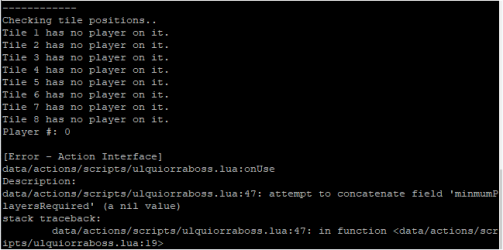Positions are correct: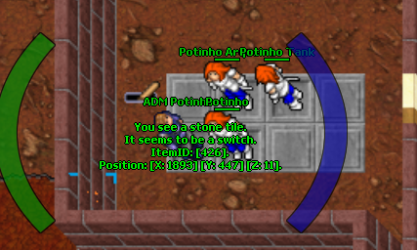All players are level 100+

Last edited:
Yeah, all players level 300+, positions are correct and no errors in console. I will test.
Post automatically merged:

Not work

View attachment 64668

Positions are correct:
View attachment 64669

All players are level 100+

try
Lua:
``````local config = {
questLevel = 100,
minmumPlayersRequired = 4,
playerPositions = {
{start = {x=1893, y=447, z=11}, destination = {x=1801, y=472, z=9}},
{start = {x=1894, y=447, z=11}, destination = {x=1802, y=472, z=9}},
{start = {x=1895, y=447, z=11}, destination = {x=1803, y=472, z=9}},
{start = {x=1896, y=447, z=11}, destination = {x=1804, y=472, z=9}},
{start = {x=1893, y=448, z=11}, destination = {x=1801, y=473, z=9}},
{start = {x=1894, y=448, z=11}, destination = {x=1802, y=473, z=9}},
{start = {x=1895, y=448, z=11}, destination = {x=1803, y=473, z=9}},
{start = {x=1896, y=448, z=11}, destination = {x=1804, y=473, z=9}}
},
spawnMonsters = {
{position = {x=1812, y=452, z=9}, monster = "Ulquiorra"}
}
}

function onUse(cid, item, frompos, item2, topos)
print("------------")
if item.uid ~= 9102 then
print("lever uniqueId is incorrect.")
print("Lever did not activate successfully.")
return true
end

print("Checking tile positions..")
local players = {}
for i = 1, #config.playerPositions do
local topPlayer = getTopCreature(config.playerPositions[i].start).uid
if topPlayer > 0 and isPlayer(topPlayer) then
if getPlayerLevel(topPlayer) >= config.questLevel then
players[#players + 1] = topPlayer
print("Tile " .. i .. "'s player (" .. getCreatureName(topPlayer) .. ") has been added to players array.")
else
print("Tile " .. i .. "'s player (" .. getCreatureName(topPlayer) .. ") does not have sufficient level.")
end
else
print("Tile " .. i .. " has no player on it.")
end
end

print("Player #: " .. #players)

if #players < config.minmumPlayersRequired then
doPlayerSendCancel(cid, "Sorry, not enough players.")
print("Not enough players to start event. (" .. #players .. "/" .. config.minimumPlayersRequired .. ")")
print("Lever did not activate successfully.")
return false
end

print("Event starting..")
print("Teleporting players..")
for i = 1, #players do
print(getCreatureName(players[i]) .. " being teleported..")
doSendMagicEffect(getThingPosition(players[i]), CONST_ME_POFF)
doTeleportThing(players[i], config.playerPositions[i].destination)
doSendMagicEffect(getThingPosition(players[i]), CONST_ME_TELEPORT)
end

print("Creating monsters..")
for i = 1, #config.spawnMonsters do
print(config.spawnMonsters[i].monster .. " being summoned..")
doSummonCreature(config.spawnMonsters[i].monster, config.spawnMonsters[i].position)
doSendMagicEffect(config.spawnMonsters[i].position, CONST_ME_TELEPORT)
end

print("Lever pulled successfully.")
return true
end``````

It should work. Did you replace it?

Lua:
``````local config = {
questLevel = 100,
minPlayers = 4,
playerPositions = {
{ start = { x = 1893, y = 447, z = 11 }, destination = { x = 1801, y = 472, z = 9 } },
{ start = { x = 1894, y = 447, z = 11 }, destination = { x = 1802, y = 472, z = 9 } },
{ start = { x = 1895, y = 447, z = 11 }, destination = { x = 1803, y = 472, z = 9 } },
{ start = { x = 1896, y = 447, z = 11 }, destination = { x = 1804, y = 472, z = 9 } }
},
spawnMonsters = {
{ position = { x = 1812, y = 452, z = 9 }, monster = "Ulquiorra" }
}
}
function onUse(cid, item, frompos, item2, topos)
if item.uid == 9102 then
local players = {}
for i = 1, #config.playerPositions do
local topPlayer = getTopCreature(config.playerPositions[i].start).uid
if topPlayer and isPlayer(topPlayer) and getPlayerLevel(topPlayer) >= config.questLevel then
players[#players + 1] = topPlayer
end
end

if #players < config.minPlayers then
doPlayerSendCancel(cid, "Sorry, not enough players.")
return false
end

for i = 1, #players do
doSendMagicEffect(getThingPosition(players[i]), CONST_ME_POFF)
doTeleportThing(players[i], config.playerPositions[i].destination)
doSendMagicEffect(getThingPosition(players[i]), CONST_ME_TELEPORT)
end

for i = 1, #config.spawnMonsters do
doSummonCreature(config.spawnMonsters[i].monster, config.spawnMonsters[i].position)
doSendMagicEffect(config.spawnMonsters[i].position, CONST_ME_TELEPORT)
end
end
return true
end``````

Last edited:
try
Lua:
``````local config = {
questLevel = 100,
minmumPlayersRequired = 4,
playerPositions = {
{start = {x=1893, y=447, z=11}, destination = {x=1801, y=472, z=9}},
{start = {x=1894, y=447, z=11}, destination = {x=1802, y=472, z=9}},
{start = {x=1895, y=447, z=11}, destination = {x=1803, y=472, z=9}},
{start = {x=1896, y=447, z=11}, destination = {x=1804, y=472, z=9}},
{start = {x=1893, y=448, z=11}, destination = {x=1801, y=473, z=9}},
{start = {x=1894, y=448, z=11}, destination = {x=1802, y=473, z=9}},
{start = {x=1895, y=448, z=11}, destination = {x=1803, y=473, z=9}},
{start = {x=1896, y=448, z=11}, destination = {x=1804, y=473, z=9}}
},
spawnMonsters = {
{position = {x=1812, y=452, z=9}, monster = "Ulquiorra"}
}
}

function onUse(cid, item, frompos, item2, topos)
print("------------")
if item.uid ~= 9102 then
print("lever uniqueId is incorrect.")
print("Lever did not activate successfully.")
return true
end

print("Checking tile positions..")
local players = {}
for i = 1, #config.playerPositions do
local topPlayer = getTopCreature(config.playerPositions[i].start).uid
if topPlayer > 0 and isPlayer(topPlayer) then
if getPlayerLevel(topPlayer) >= config.questLevel then
players[#players + 1] = topPlayer
print("Tile " .. i .. "'s player (" .. getCreatureName(topPlayer) .. ") has been added to players array.")
else
print("Tile " .. i .. "'s player (" .. getCreatureName(topPlayer) .. ") does not have sufficient level.")
end
else
print("Tile " .. i .. " has no player on it.")
end
end

print("Player #: " .. #players)

if #players < config.minmumPlayersRequired then
doPlayerSendCancel(cid, "Sorry, not enough players.")
print("Not enough players to start event. (" .. #players .. "/" .. config.minimumPlayersRequired .. ")")
print("Lever did not activate successfully.")
return false
end

print("Event starting..")
print("Teleporting players..")
for i = 1, #players do
print(getCreatureName(players[i]) .. " being teleported..")
doSendMagicEffect(getThingPosition(players[i]), CONST_ME_POFF)
doTeleportThing(players[i], config.playerPositions[i].destination)
doSendMagicEffect(getThingPosition(players[i]), CONST_ME_TELEPORT)
end

print("Creating monsters..")
for i = 1, #config.spawnMonsters do
print(config.spawnMonsters[i].monster .. " being summoned..")
doSummonCreature(config.spawnMonsters[i].monster, config.spawnMonsters[i].position)
doSendMagicEffect(config.spawnMonsters[i].position, CONST_ME_TELEPORT)
end

print("Lever pulled successfully.")
return true
end``````
Works with 4 players, but when got 3, console gives error, like bellow: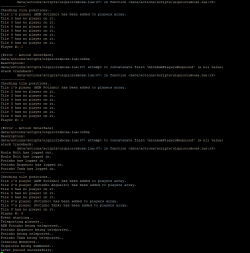Post automatically merged:

It should work. Did you replace it?

Lua:
``````local config = {
questLevel = 100,
playerPositions = {
{ start = { x = 1893, y = 447, z = 11 }, destination = { x = 1801, y = 472, z = 9 } },
{ start = { x = 1894, y = 447, z = 11 }, destination = { x = 1802, y = 472, z = 9 } },
{ start = { x = 1895, y = 447, z = 11 }, destination = { x = 1803, y = 472, z = 9 } },
{ start = { x = 1896, y = 447, z = 11 }, destination = { x = 1804, y = 472, z = 9 } }
},
spawnMonsters = {
{ position = { x = 1812, y = 452, z = 9}, monster = "Ulquiorra" }
}
}
function onUse(cid, item, frompos, item2, topos)
if item.uid == 9102 then
local players = {}
for i = 1, #config.playerPositions do
local topPlayer = getTopCreature(config.playerPositions[i].start)
if topPlayer and isPlayer(topPlayer) and getPlayerLevel(topPlayer) >= config.questLevel then
players[#players + 1] = topPlayer.uid
end
end

if #players == 0 then
doPlayerSendCancel(cid, "Sorry, not possible.")
return false
end

for i = 1, #players do
doSendMagicEffect(getThingPosition(players[i]), CONST_ME_POFF)
doTeleportThing(players[i], config.playerPositions[i].destination)
doSendMagicEffect(getThingPosition(players[i]), CONST_ME_TELEPORT)
end

for i = 1, #config.spawnMonsters do
doSummonCreature(config.spawnMonsters[i].monster, config.spawnMonsters[i].position)
doSendMagicEffect(config.spawnMonsters[i].position, CONST_ME_TELEPORT)
end
end
return true
end``````
yeah, have replaced and nothing happens

Works with 4 players, but when got 3, console gives error, like bellow:

View attachment 64671
Post automatically merged:

yeah, have replaced and nothing happens
alright, here you go. should work now.
Lua:
``````local config = {
questLevel = 100,
minimumPlayersRequired = 4,
playerPositions = {
{start = {x=1893, y=447, z=11}, destination = {x=1801, y=472, z=9}},
{start = {x=1894, y=447, z=11}, destination = {x=1802, y=472, z=9}},
{start = {x=1895, y=447, z=11}, destination = {x=1803, y=472, z=9}},
{start = {x=1896, y=447, z=11}, destination = {x=1804, y=472, z=9}},
{start = {x=1893, y=448, z=11}, destination = {x=1801, y=473, z=9}},
{start = {x=1894, y=448, z=11}, destination = {x=1802, y=473, z=9}},
{start = {x=1895, y=448, z=11}, destination = {x=1803, y=473, z=9}},
{start = {x=1896, y=448, z=11}, destination = {x=1804, y=473, z=9}}
},
spawnMonsters = {
{position = {x=1812, y=452, z=9}, monster = "Ulquiorra"}
}
}

function onUse(cid, item, frompos, item2, topos)
print("------------")
if item.uid ~= 9102 then
print("lever uniqueId is incorrect.")
print("Lever did not activate successfully.")
return true
end

print("Checking tile positions..")
local players = {}
for i = 1, #config.playerPositions do
local topPlayer = getTopCreature(config.playerPositions[i].start).uid
if topPlayer > 0 and isPlayer(topPlayer) then
if getPlayerLevel(topPlayer) >= config.questLevel then
players[#players + 1] = topPlayer
print("Tile " .. i .. "'s player (" .. getCreatureName(topPlayer) .. ") has been added to players array.")
else
print("Tile " .. i .. "'s player (" .. getCreatureName(topPlayer) .. ") does not have sufficient level.")
end
else
print("Tile " .. i .. " has no player on it.")
end
end

print("Player #: " .. #players)

if #players < config.minimumPlayersRequired then
doPlayerSendCancel(cid, "Sorry, not enough players.")
print("Not enough players to start event. (" .. #players .. "/" .. config.minimumPlayersRequired .. ")")
print("Lever did not activate successfully.")
return false
end

print("Event starting..")
print("Teleporting players..")
for i = 1, #players do
print(getCreatureName(players[i]) .. " being teleported..")
doSendMagicEffect(getThingPosition(players[i]), CONST_ME_POFF)
doTeleportThing(players[i], config.playerPositions[i].destination)
doSendMagicEffect(getThingPosition(players[i]), CONST_ME_TELEPORT)
end

print("Creating monsters..")
for i = 1, #config.spawnMonsters do
print(config.spawnMonsters[i].monster .. " being summoned..")
doSummonCreature(config.spawnMonsters[i].monster, config.spawnMonsters[i].position)
doSendMagicEffect(config.spawnMonsters[i].position, CONST_ME_TELEPORT)
end

print("Lever pulled successfully.")
return true
end``````

alright, here you go. should work now.
Lua:
``````local config = {
questLevel = 100,
minimumPlayersRequired = 4,
playerPositions = {
{start = {x=1893, y=447, z=11}, destination = {x=1801, y=472, z=9}},
{start = {x=1894, y=447, z=11}, destination = {x=1802, y=472, z=9}},
{start = {x=1895, y=447, z=11}, destination = {x=1803, y=472, z=9}},
{start = {x=1896, y=447, z=11}, destination = {x=1804, y=472, z=9}},
{start = {x=1893, y=448, z=11}, destination = {x=1801, y=473, z=9}},
{start = {x=1894, y=448, z=11}, destination = {x=1802, y=473, z=9}},
{start = {x=1895, y=448, z=11}, destination = {x=1803, y=473, z=9}},
{start = {x=1896, y=448, z=11}, destination = {x=1804, y=473, z=9}}
},
spawnMonsters = {
{position = {x=1812, y=452, z=9}, monster = "Ulquiorra"}
}
}

function onUse(cid, item, frompos, item2, topos)
print("------------")
if item.uid ~= 9102 then
print("lever uniqueId is incorrect.")
print("Lever did not activate successfully.")
return true
end

print("Checking tile positions..")
local players = {}
for i = 1, #config.playerPositions do
local topPlayer = getTopCreature(config.playerPositions[i].start).uid
if topPlayer > 0 and isPlayer(topPlayer) then
if getPlayerLevel(topPlayer) >= config.questLevel then
players[#players + 1] = topPlayer
print("Tile " .. i .. "'s player (" .. getCreatureName(topPlayer) .. ") has been added to players array.")
else
print("Tile " .. i .. "'s player (" .. getCreatureName(topPlayer) .. ") does not have sufficient level.")
end
else
print("Tile " .. i .. " has no player on it.")
end
end

print("Player #: " .. #players)

if #players < config.minimumPlayersRequired then
doPlayerSendCancel(cid, "Sorry, not enough players.")
print("Not enough players to start event. (" .. #players .. "/" .. config.minimumPlayersRequired .. ")")
print("Lever did not activate successfully.")
return false
end

print("Event starting..")
print("Teleporting players..")
for i = 1, #players do
print(getCreatureName(players[i]) .. " being teleported..")
doSendMagicEffect(getThingPosition(players[i]), CONST_ME_POFF)
doTeleportThing(players[i], config.playerPositions[i].destination)
doSendMagicEffect(getThingPosition(players[i]), CONST_ME_TELEPORT)
end

print("Creating monsters..")
for i = 1, #config.spawnMonsters do
print(config.spawnMonsters[i].monster .. " being summoned..")
doSummonCreature(config.spawnMonsters[i].monster, config.spawnMonsters[i].position)
doSendMagicEffect(config.spawnMonsters[i].position, CONST_ME_TELEPORT)
end

print("Lever pulled successfully.")
return true
end``````
Works, thanks Xiki! To remove the logs, just delete "print" lines, right?

Works, thanks Xiki! To remove the logs, just delete "print" lines, right?
correct for the most part. xP
Lua:
``````local config = {
questLevel = 100,
minimumPlayersRequired = 4,
playerPositions = {
{start = {x=1893, y=447, z=11}, destination = {x=1801, y=472, z=9}},
{start = {x=1894, y=447, z=11}, destination = {x=1802, y=472, z=9}},
{start = {x=1895, y=447, z=11}, destination = {x=1803, y=472, z=9}},
{start = {x=1896, y=447, z=11}, destination = {x=1804, y=472, z=9}},
{start = {x=1893, y=448, z=11}, destination = {x=1801, y=473, z=9}},
{start = {x=1894, y=448, z=11}, destination = {x=1802, y=473, z=9}},
{start = {x=1895, y=448, z=11}, destination = {x=1803, y=473, z=9}},
{start = {x=1896, y=448, z=11}, destination = {x=1804, y=473, z=9}}
},
spawnMonsters = {
{position = {x=1812, y=452, z=9}, monster = "Ulquiorra"}
}
}

function onUse(cid, item, frompos, item2, topos)
if item.uid ~= 9102 then
return true
end

local players = {}
for i = 1, #config.playerPositions do
local topPlayer = getTopCreature(config.playerPositions[i].start).uid
if topPlayer > 0 and isPlayer(topPlayer) and getPlayerLevel(topPlayer) >= config.questLevel then
players[#players + 1] = topPlayer
end
end

if #players < config.minimumPlayersRequired then
doPlayerSendCancel(cid, "Sorry, not enough players.")
return false
end

for i = 1, #players do
doSendMagicEffect(getThingPosition(players[i]), CONST_ME_POFF)
doTeleportThing(players[i], config.playerPositions[i].destination)
doSendMagicEffect(getThingPosition(players[i]), CONST_ME_TELEPORT)
end

for i = 1, #config.spawnMonsters do
doSummonCreature(config.spawnMonsters[i].monster, config.spawnMonsters[i].position)
doSendMagicEffect(config.spawnMonsters[i].position, CONST_ME_TELEPORT)
end
return true
end``````

•Alberto Cabrera

Replies
2
Views
270
Replies
1
Views
435
Replies
2
Views
244
Replies
0
Views
257
Replies
8
Views
748
Replies
5
Views
728